﻿ Subtract
Liquid Studio Documentation
Data Mapping / Data Mapper Functions / By Category / Maths / Subtract
In This Topic
Subtract
In This Topic

Subtract
Maths
##### Icon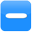##### Description
Subtract one numeric value from another
##### Inputs
 Minuend Value to subtract from Subtrahend Value to subtract
##### Outputs
 Result Result of subtracting second input from first
##### Properties
 None -

##### Usage

The Data Mapper Maths Subtract function allows you to carry out subtraction on input values. You can use the Subtract function with input source data or in conjunction with other arithmetic functions in the Maths section. To apply the Maths Subtract function, use the following process:

Create a new Data Mapper file, dragging your XML data source and targets into the editor area. For this example we are using payroll_source.xsd, an XML Schema Definition inferred from the following source:

Source XML
Copy Code
```<payroll>
<employee payroll_id="126">
<rate>9.45</rate>
<hours>35</hours>
<bonus>50.00</bonus>
<funding>350</funding>
</employee>
<employee payroll_id="129">
<rate>10.50</rate>
<hours>40</hours>
<bonus>150.00</bonus>
<funding>500</funding>
</employee>
<employee payroll_id="130">
<rate>8.90</rate>
<hours>35</hours>
<bonus>100.00</bonus>
<funding>500</funding>
</employee>
<employee payroll_id="133">
<rate>11.20</rate>
<hours>40</hours>
<bonus>200.00</bonus>
<funding>600</funding>
</employee>
</payroll>
```

For our target schema we will be using payroll_target.xsd, inferred from the following XML:

Target XML
Copy Code
```<wages>
<payee>
<ref>122</ref>
<result type="balance">50.00</result>
</payee>
<payee>
<ref>125</ref>
<result type="balance">47.50</result>
</payee>
<payee>
<ref>126</ref>
<result type="balance">60.30</result>
</payee>
</wages>
```

The data models payroll data for temporary staff within an organisation. The output is designed to capture some value calculated from the source, using the "type" attribute to indicate what the calculation result represents. Let's assume that we want to calculate the balance after the cost of employing each staff member. To calculate this we will need to subtract the weekly wage plus bonus for an employee from the funding allocated to them. We will need to carry out multiplication and addition calculations to work out the weekly wage before we can subtract it, so our final mapping process will involve a few functions working in conjunction with one another. Here is the Data Mapper with Source and Target imported:Drag the Maths Subtract function from the Component Palette into the mapping area: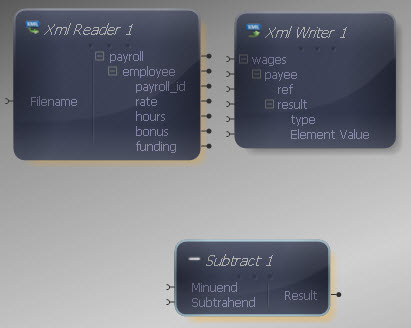The Subtract component has two inputs, representing the value to subtract from and the value to subtract. Both inputs and output of the Subtract function may be connected to items in the XML Reader/ Writer or other components in the Mapper.

Connect the first Subtract input to the "funding" output in the XML Reader.For the second Subtract input, we need to carry out another couple of calculations. Drag an Add function onto the Mapper, connecting its first input to the "bonus" output in the XML Reader.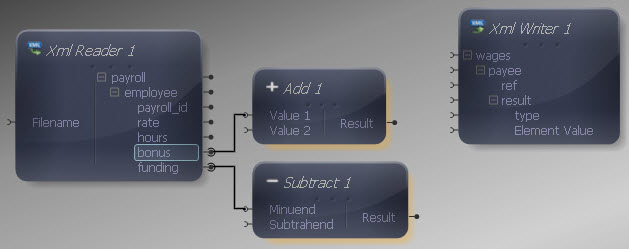Now drag a Multiply function onto the Mapper, connecting its inputs to the "rate" and "hours" outputs in the XML Reader.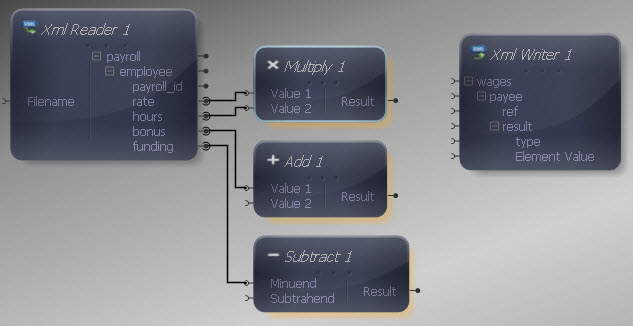Connect the output of the Multiply function to the second input in the Add function. Then connect the output of the Add function to the second input in the Subtract function.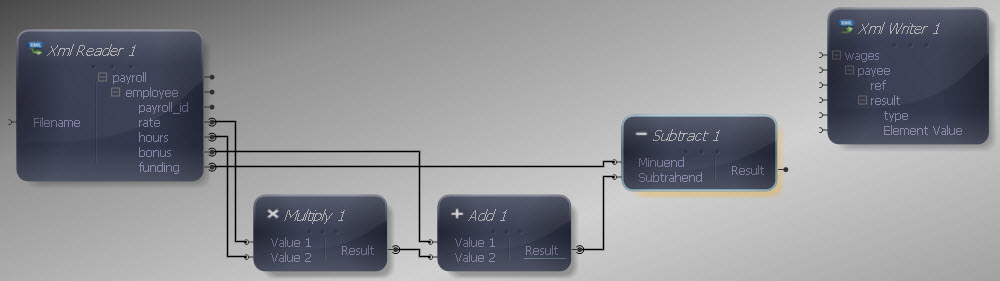Now connect the Subtract output to the Element Value input in the XML Writer, so that the addition result is written into the "result" element.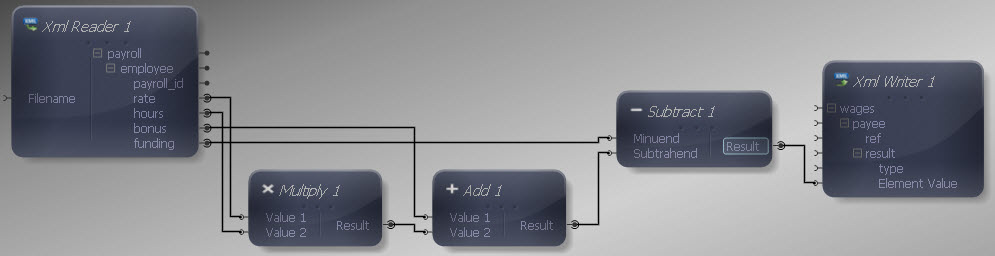Now we need to include the "type" attribute in the source, so add a Constant Value from the Data Type section. Right-click it and choose Show Properties. Choose a String Data Type and enter "balance" as the value.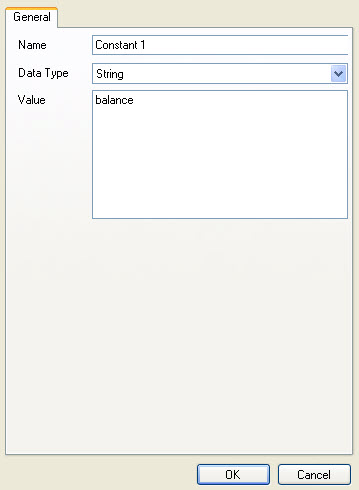Connect the Constant output to the "type" input in the XML Writer.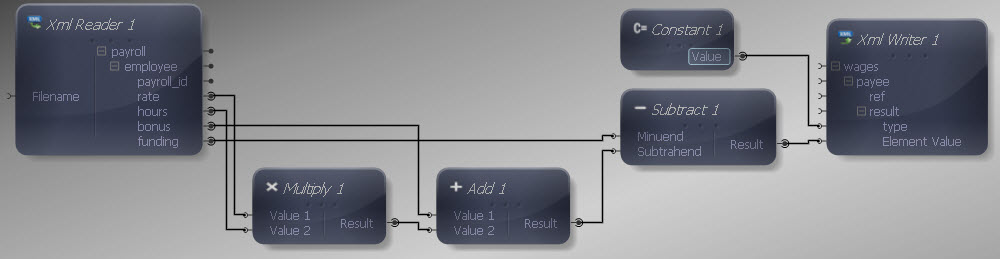Finally let's make the remaining input and output connections. We do not want to map all data items in the source, as we are only interested in certain values. Map "payroll" to "wages", "employee" to "payee" and "payroll_id" to "ref". We don't need to map anything to the "result" element as it will be written out each time the Mapper maps the addition result to it. Although we have used the remaining input values for calculations, we do not need to map them individually to the target, as we only want the calculation result.We can now execute the transform by pressing Shift-F5 or the Execute button (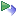). The transform is applied and the file we selected as output opens in the editor:

Output XML
Copy Code
```<wages>
<payee>
<ref>126</ref>
<result type="balance">-30.75</result>
</payee>
<payee>
<ref>129</ref>
<result type="balance">-70.00</result>
</payee>
<payee>
<ref>130</ref>
<result type="balance">88.50</result>
</payee>
<payee>
<ref>133</ref>
<result type="balance">-48.00</result>
</payee>
</wages>
```

The output contains a positive or negative indicator of the balance after employing each staff member.

This is a scalar function so the standard rules apply if multiple values are presented to one or more inputs.